﻿

### Generalizations of Fibonacci and Lucas sequences

#### Abstract

In this paper, we consider the Hecke groups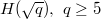$H(\sqrt{q}),~q\geq 5$ prime number, and we find an interesting number sequence whichis denoted by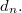$d_n.$ For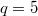$q=5$, we get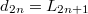$d_{2n}=L_{2n+1}$ and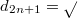$d_{2n+1}=\sqrt{%5}F_{2n+2}$ where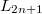$L_{2n+1}$ is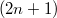$(2n+1)$th Lucas number and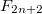$F_{2n+2}$ is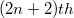$(2n +2)th$ Fibonacci number. From this sequence, we obtain two new sequenceswhich are, in a sense, generalizations of Fibonacci and Lucas sequences.

DOI Code: 10.1285/i15900932v21n1p113

Keywords: Hecke groups; Fibonacci numbers; Lucas numbers

Classification: 11B39; 20H10

Full Text: PDFThis work is licensed under a Creative Commons Attribuzione - Non commerciale - Non opere derivate 3.0 Italia License.